2021 NBA Rookie of the Year Odds
+250
2.5 to 1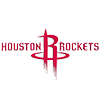Jalen Green
19.1% implied probability

+300
3 to 1Cade Cunningham
16.7% implied probability

+800
8 to 1Jalen Suggs
7.4% implied probability

+800
8 to 1Evan Mobley
7.4% implied probability

+1200
12 to 1Chris Duarte
5.1% implied probability

+1200
12 to 1Scottie Barnes
5.1% implied probability

+1400
14 to 1Alperen Sengun
4.5% implied probability

+1800
18 to 1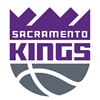Davion Mitchell
3.5% implied probability

No changes have been recorded yet.
+2000
20 to 1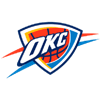Josh Giddey
3.2% implied probability

+3500
35 to 1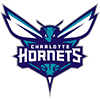James Bouknight
1.9% implied probability

+3500
35 to 1Jonathan Kuminga
1.9% implied probability

+4000
40 to 1Keon Johnson
1.6% implied probability

No changes have been recorded yet.
+4000
40 to 1Franz Wagner
1.6% implied probability

+4000
40 to 1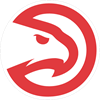Jalen Johnson
1.6% implied probability

+4500
45 to 1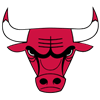Ayo Dosunmu
1.5% implied probability

No changes have been recorded yet.
+4500
45 to 1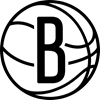Cam Thomas
1.5% implied probability

No changes have been recorded yet.
+4500
45 to 1Tre Mann
1.5% implied probability

+4500
45 to 1Moses Moody
1.5% implied probability

No changes have been recorded yet.
+4500
45 to 1Nah'Shon Hyland
1.5% implied probability

No changes have been recorded yet.
+5000
50 to 1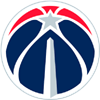Corey Kispert
1.3% implied probability

+6000
60 to 1Sharife Cooper
1.1% implied probability

+6000
60 to 1Trey Murphy
1.1% implied probability

+6500
65 to 1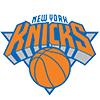Quentin Grimes
1.0% implied probability

No changes have been recorded yet.
+6500
65 to 1Ziaire Williams
1.0% implied probability

+8000
80 to 1Usman Garuba
0.8% implied probability

No changes have been recorded yet.
+8000
80 to 1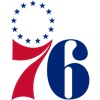Jaden Springer
0.8% implied probability

+8000
80 to 1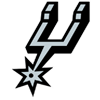Josh Primo
0.8% implied probability

No changes have been recorded yet.
+8000
80 to 1Miles McBride
0.8% implied probability

No changes have been recorded yet.
+8000
80 to 1Kai Jones
0.8% implied probability

+8000
80 to 1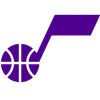Jared Butler
0.8% implied probability

No changes have been recorded yet.
+9000
90 to 1Isaiah Jackson
0.7% implied probability

No changes have been recorded yet.
+15000
150 to 1Luka Garza
0.4% implied probability

No changes have been recorded yet.
+15000
150 to 1Jock Landale
0.4% implied probability

No changes have been recorded yet.